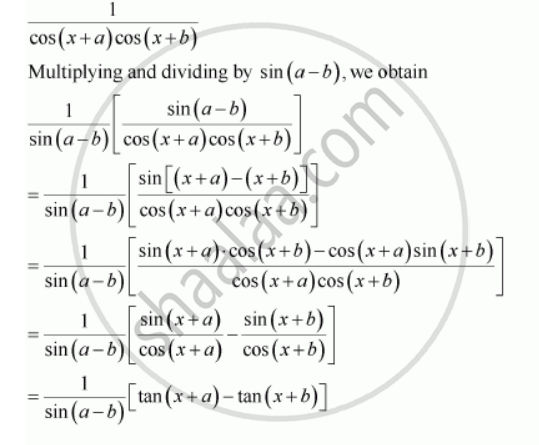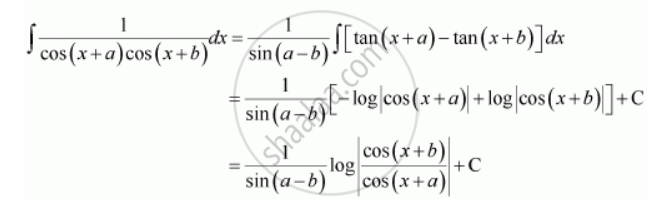Share

# Integrate the Functions 1/(Cos (X+A) Cos(X+B)) - Mathematics

#### Question

Integrate the functions 1/(cos (x+a) cos(x+b))

#### SolutionIs there an error in this question or solution?

#### Video TutorialsVIEW ALL 

Solution Integrate the Functions 1/(Cos (X+A) Cos(X+B)) Concept: Integration as an Inverse Process of Differentiation.
S